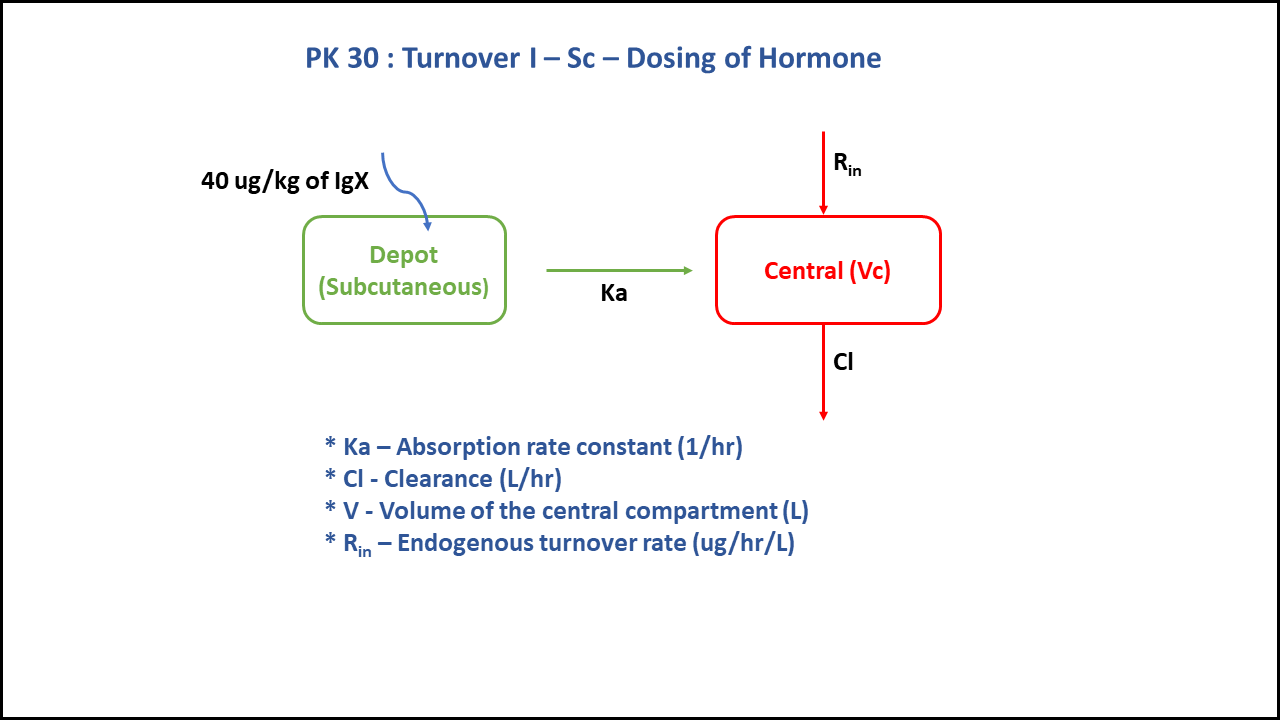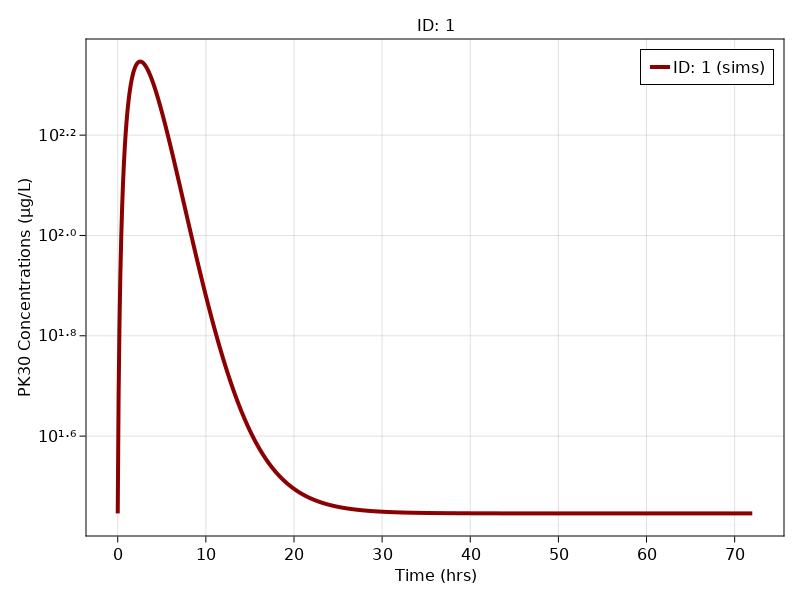# Exercise PK30 - Turnover I - SC - Dosing of hormone

### Background

• Structural model - One compartment first order elimination with zero order production of hormone

• Route of administration - Subcutaneous

• Dosage Regimen - 40 mcg/kg

• Number of Subjects - 1### Learning Outcome

With subcutaneous dose information, we learn to discriminate between the clearance and the rate of synthesis. Further simplification of the model is done by assuming bioavailability is 100% and concentration of the endogenous compound equals concentration at baseline (turnover/clearance)

### Objectives

In this tutorial, you will learn how to build a one compartment PK turnover model, following first order elimination kinetics and zero order hormone production.

### Libraries

Call the "necessary" libraries to get started

using Random
using Pumas
using PumasUtilities
using CairoMakie


### Model

In this two compartment model, we administer dose subcutaneously.

pk_30           = @model begin
desc        = "Two Compartment Model"
timeu       = u"hr"
end

@param begin
"Absorption rate constant (hr⁻¹)"
tvka        ∈ RealDomain(lower=0)
"Clearance (L/kg/hr)"
tvcl        ∈ RealDomain(lower=0)
"Turnover Rate (hr⁻¹)"
tvsynthesis ∈ RealDomain(lower=0)
"Volume of Central Compartment (L/kg)"
tvv         ∈ RealDomain(lower=0)
Ω           ∈ PDiagDomain(4)
end

@random begin
η           ~ MvNormal(Ω)
end

@pre begin
Ka          = tvka * exp(η)
Cl          = tvcl * exp(η)
Synthesis   = tvsynthesis * exp(η)
V           = tvv * exp(η)
end

@init begin
Central     = Synthesis/(Cl/V) # Concentration at Baseline = Turnover Rate (0.78) / Cl of hormone (0.028)
end

@dynamics begin
Depot'      = -Ka * Depot
Central'    =  Ka * Depot + Synthesis - (Cl/V) * Central
end

@derived begin
cp          = @. Central/V
"""
Observed Concentration (mcg/L)
"""
end
end

PumasModel
Parameters: tvka, tvcl, tvsynthesis, tvv, Ω, σ_add
Random effects: η
Covariates:
Dynamical variables: Depot, Central
Derived: cp, dv
Observed: cp, dv


### Parameters

The parameters are as given below. tv represents the typical value for parameters.

• $Ka$ - Absorption rate constant (hr⁻¹)

• $Cl$ - Clearance (L/kg/hr)

• $Synthesis$ - Turnover Rate (hr⁻¹)

• $V$ - Volume of Central Compartment (L/kg)

• $Ω$ - Between Subject Variability

• $σ$ - Residual error

param =  ( tvka         = 0.539328,
tvcl         = 0.0279888,
tvsynthesis  = 0.781398,
tvv          = 0.10244,
Ω            = Diagonal([0.0,0.0,0.0,0.0]),

(tvka = 0.539328, tvcl = 0.0279888, tvsynthesis = 0.781398, tvv = 0.10244,
Ω = [0.0 0.0 0.0 0.0; 0.0 0.0 0.0 0.0; 0.0 0.0 0.0 0.0; 0.0 0.0 0.0 0.0], σ


### Dosage Regimen

A dose of 40 mcg/kg is given subcutaneously to a Single subject.

ev1  = DosageRegimen(40,time = 0, cmt = 1)
sub1 = Subject(id = 1, events = ev1)

Subject
ID: 1
Events: 1


### Simulation

To simulate plasma concentration with turnover rate after oral administration.

Random.seed!(1234)
sim_sub1 = simobs(pk_30, sub1, param, obstimes = 0:0.1:72)


### Visualization

f, a, p = sim_plot(pk_30, [sim_sub1],
observations = :cp,
color = :redsblues,
linewidth = 4,
axis = (xlabel = "Time (hrs)",
ylabel = "PK30 Concentrations (μg/L)",
xticks = 0:10:80, yscale = log10))
axislegend(a)
f